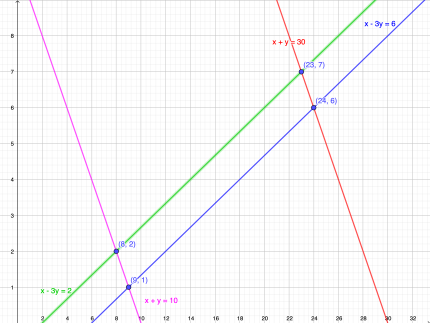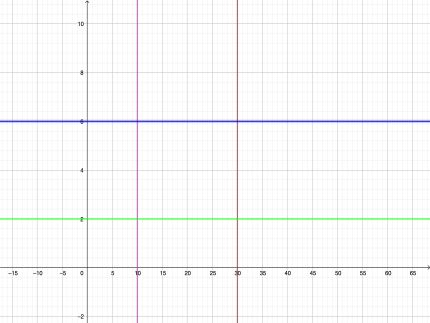# Use a change of variables to find the volume of the solid region lying below the surface f (x,...

## Question:

Use a change of variables to find the volume of the solid region lying below the surface {eq}\displaystyle f (x, y) = (x - 3y)^{2} \sqrt{ x + y} {/eq} and above the parallelogram in the xy-plane with Vertices {eq}(9,1), (8,2), (24,6) {/eq} and {eq}(23,7). {/eq}

## Double integrals:

Let {eq}f(x,y) {/eq} be a function of two variables such that {eq}f(x,y)\geq 0\ \text{for all }(x,y)\in R,\ \text{where} \ R {/eq} is a bounded region in the {eq}xy {/eq}-plane. The double integral

{eq}\displaystyle\iint_{R}f(x,y)dxdy, {/eq}

represent the volume of the solid region between the surface {eq}z=f(x,y) {/eq} and the region {eq}R\ \text{in the}\ xy {/eq}-plane,

The volume of the solid region lying under the surface {eq}f(x,y) {/eq} is given by the double integral

{eq}\displaystyle\iint_{R}f(x,y)dxdy {/eq}

where {eq}R {/eq} is the projection of the surface in the {eq}xy {/eq} plane. In this case, we have that {eq}R {/eq} is a parallelogram in the {eq}xy {/eq}-plane with vertices

{eq}(9,1),\ (8,2),\ (24,6),\ (23,7) {/eq}}Let's consider the following change of variables

{eq}\left\{\begin{array}{l} u=x+y \\ v=x-3 y \end{array} \quad\equiv\quad\left\{\begin{array}{l} x=\frac{1}{4}(3 u+v) \\ y=\frac{1}{4}(u-v) \end{array}\right.\right. {/eq}

The given function can be written as

{eq}f(u,v)=f(x(u, v), y(u, v))=v^{2} \sqrt{u} {/eq}

and the volume element result

{eq}dA=d x d y=|J| d u d v {/eq}

where {eq}J {/eq} is the jacobian

{eq}J=\left|\begin{array}{ll} \frac{\partial x}{\partial u} & \frac{\partial x}{\partial v} \\ \frac{\partial y}{\partial u} & \frac{\partial y}{\partial v} \end{array}\right|=\left|\begin{array}{cc} \frac{3}{4} & \frac{1}{4} \\ \frac{1}{4} & -\frac{1}{4} \end{array}\right|=-\frac{1}{4} {/eq}

Taking into account that

{eq}\begin{aligned} &10 \leq u=x+y \leq 30\\ &2 \leq v=x-3 y \leq 6 \end{aligned} {/eq}

we notice that the parallelogram is mapped as the rectangle

{eq}R_{u v}=\{(u, v) | 10 \leq u \leq 30\ \text { and }\ 2 \leq v \leq 6\} {/eq}

in {eq}u,v {/eq} coordinates.The given integral result:

{eq}\begin{aligned} \iint_{R} f(x, y) d y d x &=\int_{10}^{30} \int_{2}^{6} v^{2} \sqrt{u} \frac{1}{4} d v d u \\ &=\frac{1}{4} \int_{10}^{30} \sqrt{u} d u \int_{2}^{6} v^{2} d v \\ &x=\frac{1}{4} \frac{2}{3} \left[x^{3 / 2}\right]_{10}^{30}\left[\frac{v^{3}}{3}\right]_{2}^{6} \\ &=\frac{1040}{9} \sqrt{10}(3 \sqrt{3}-1)(\text { units })^{3} \\ & \approx 1533.35 \text { (units) }^{3} \end{aligned} {/eq}